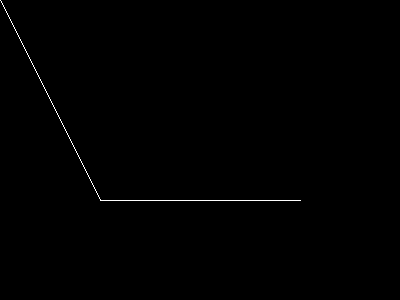PHP 8.1.24 Released!

# imageopenpolygon

(PHP 7 >= 7.2.0, PHP 8)

imageopenpolygonオープンポリゴンを描画する

### 説明

PHP 8.0.0 以降のシグネチャ (名前付き引数をサポートしていません)

imageopenpolygon(GdImage `\$image`, array `\$points`, int `\$color`): bool

imageopenpolygon(
GdImage `\$image`,
array `\$points`,
int `\$num_points`,
int `\$color`
): bool

imageopenpolygon() 関数は、 与えられた `image` のオープンポリゴンを描画します。 imagepolygon() とは異なり、 始点と終点の間の線は描かれません。

### パラメータ

`image`

imagecreatetruecolor()のような画像作成関数が返す GdImage オブジェクト。

`points`

ポリゴンの点を含む配列:

 points = x0 points = y0 points = x1 points = y1

`num_points`

`color`

imagecolorallocate() で作成された色識別子。

### 変更履歴

バージョン 説明
8.1.0 引数 `num_points` は、推奨されなくなりました。
8.0.0 `image` は、 GdImage クラスのインスタンスを期待するようになりました。 これより前のバージョンでは、有効な `gd` resource が期待されていました。

### 例

`<?php// 空の画像を生成します。\$image = imagecreatetruecolor(400, 300);// 色をポリゴンに割り当てます。\$col_poly = imagecolorallocate(\$image, 255, 255, 255);// ポリゴンを描画します。imageopenpolygon(\$image, array( 0, 0, 100, 200, 300, 200 ), 3, \$col_poly);// 画像をブラウザに出力します。header('Content-type: image/png');imagepng(\$image);imagedestroy(\$image);?>`### 参考

`In case you want to use an open polygon but are stuck with a PHP version prior to 7.2, a solution may be to 'backplot' your array to its original start. Say you have an array of pixels (below seperated by commas)<?php\$arr = array();for (\$i = 0; \$i < count(\$pixels); \$i++) { \$pixel = explode(',', \$pixels[\$i]); if ((\$pixel > 0) && (\$pixel > 0)) { \$arr[] = \$pixel; \$arr[] = \$pixel; }}imagepolygon(\$im, \$arr, (count(\$arr) / 2), \$otcolor);?>you can replace this by something like<?php\$arr = array();for (\$i = 0; \$i < count(\$pixels); \$i++) { \$pixel = explode(',', \$pixels[\$i]); \$arr[] = \$pixel; \$arr[] = \$pixel;}// imageopenpolygon(\$im, \$arr, (count(\$arr) / 2), \$otcolor) is not possible, so...for (\$i = (count(\$pixels)-1); \$i >= 0; \$i--) { \$pixel = explode(',', \$pixels[\$i]); \$arr[] = \$pixel; \$arr[] = \$pixel;}imagepolygon(\$im, \$arr, (count(\$arr) / 2), \$otcolor);?>`